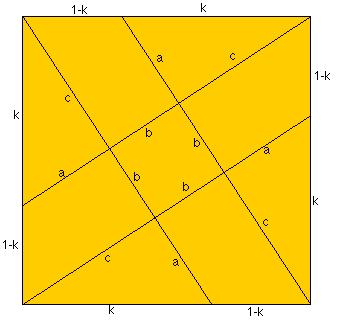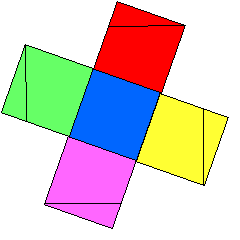#### You may also like### Square Pegs

Which is a better fit, a square peg in a round hole or a round peg in a square hole?### Not So Little X

Two circles are enclosed by a rectangle 12 units by x units. The distance between the centres of the two circles is x/3 units. How big is x?### Lying and Cheating

Follow the instructions and you can take a rectangle, cut it into 4 pieces, discard two small triangles, put together the remaining two pieces and end up with a rectangle the same size. Try it!

# Square Areas

##### Age 11 to 14Challenge LevelThe area of the inner square has area of 0.2 square units. First draw the original square, then remove the triangles. The triangles can then be added to the trapeziums to make four equal squares. This gives a total of five equal squares which have a total area of 1 square unit. Therefore each of the smaller squares must have an area of 0.2 square units.

If we don't use the midpoints on the sides of the big square the problem can be generalised by considering the lengths as shown in the diagram. In the above solution k = 1/2 and a = b .

By enlargement (or similar triangles) c = ka .

Using Pythagoras Theorem twice we get $a^2 + c^2 = k^2$ and also $(a + b + c)^2 = 1 + k^2$

By some routine algebra we get $a^2 = k^2/(1 + k^2)$ and the area of the inner square is $b^2 = (1 - k)^2/(1 + k^2).$